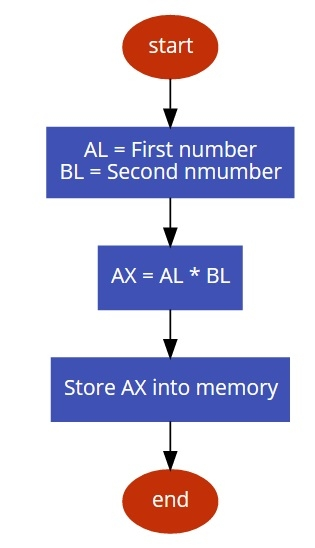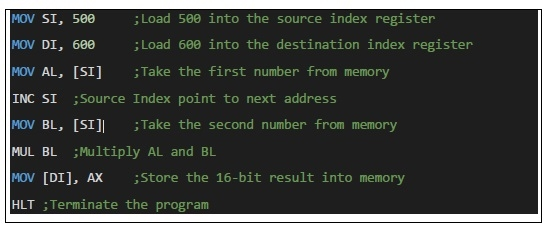# 8086 program to multiply two 8-bit numbers

In this program we will see how to multiply two 8-bit numbers.

## Problem Statement

Write 8086 Assembly language program to multiply two 8-bit numbers stored in memory address offset 500 and 501.

## Discussiont

In 8086 there is MUL instruction. So the task is too simple. Here we are taking the numbers from memory and after that performing the multiplication operation. As 8-bit numbers are taken, after multiplication AX (16-bit) will store the result.

Data

500
99
501
25

## Flow Diagram## Program